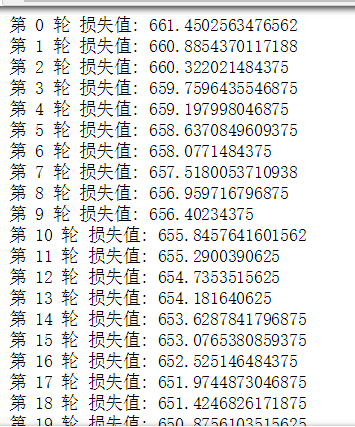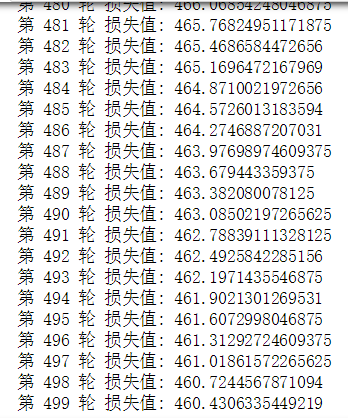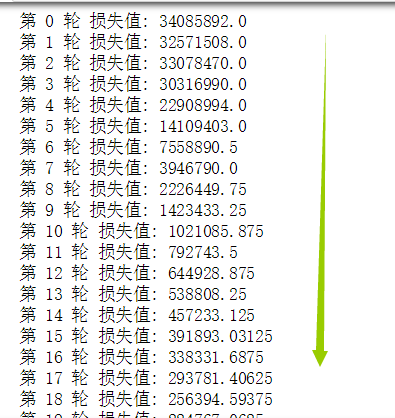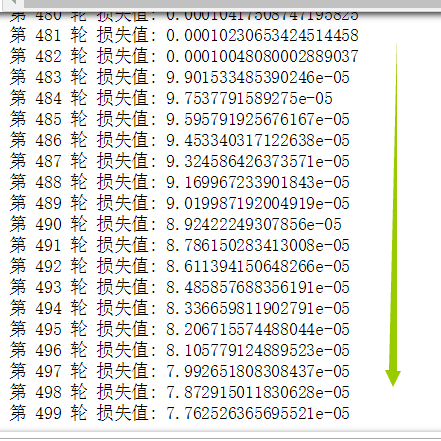## 学习笔记 (3.4)

model=torch.nn.Sequential(
torch.nn.Linear(D_in,H),
torch.nn.ReLU(),
torch.nn.Linear(H,D_out),
)

#这次我用使用Pytorch中的nn库来创建神经网络，用Pytorch autograd来构建计算图和gradients然后Pytorch会帮我们自动计算gradients
#nn==neural network,在backward的基础上让网络变的更简单
import torch.nn as nn
import torch
N,D_in,H,D_out=64,1000,100,10
x=torch.randn(N,D_in)
y=torch.randn(N,D_out)
model=torch.nn.Sequential(##模型的组成顺序
#第一层是一个线性结构 这里和前面有点不一样了这里有偏置的
#y=w1*x+b1
torch.nn.Linear(D_in,H),
#第二层是一个ReLU激活函数
torch.nn.ReLU(),
#第三层也是一个线性的结构和第一层一样的
torch.nn.Linear(H,D_out),
)
#这是把w1，w2输入层-->隐藏层-->输出层的权重初始化为normal类型的
#关于normal，官网的解释是 ：Returns a Tensor of random numbers drawn from separate normal distributions who’s mean and standard deviation are given.
#大概是返回一个张量，张量里面的随机数是从相互独立的正态分布中随机生成的，这样使得权重会好一点，训练效果也好，过一会我会做实验
torch.nn.init.normal_(model.weight)
torch.nn.init.normal_(model.weight)

#这里使用了nn里的MSELoss来处理loss损失数值，里面的参数reduction
#reduction = ‘none’，直接返回向量形式的 loss
#reduction = ‘sum’，返回loss之和
#reduction = ''elementwise_mean，返回loss的平均值
#reduction = ''mean，返回loss的平均值
loss_fn=nn.MSELoss(reduction='sum')
learning_rate= 1e-6# 1*10^6

for it in range(500):
y_pred=model(x)
loss=loss_fn(y_pred,y)
print("第",it,"轮","损失值:",loss.item())
loss.backward()
for param in model.parameters():



### 我们将把权重正态分布随机化的语句注释掉，跑一下代码，观察网络训练情况：### 我们添加上这一句：reduction = ‘none’，直接返回向量形式的 loss
reduction = ‘sum’，返回loss之和
reduction = ''elementwise_mean，返回loss的平均值
reduction = ''mean，返回loss的平均值

posted on 2020-03-05 23:42  那我先走了  阅读(163)  评论(0编辑  收藏  举报